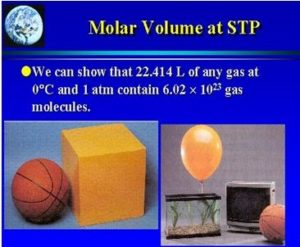# Limiting reactant of Chemical Reaction , Mole and volume of gases

Every chemical reaction needs accurately calculated amounts of reactants to obtain the required amounts of products, If the amount of one of the reactants increases than required, this extra amount remains as it is, without participation in the reaction, The other reactant is completely consumed and determines the quantities of products formed and it is known as the limiting reactant.

### Limiting reactant

Limiting reactant is the reactant which is completely consumed during the chemical reaction and reacts with other reactants to produce the lowest number of moles of the product .

#### The mole and volume of gases

The solid or liquid matter has a definite and constant volume , It can be measured by various methods , On the other hand , the volume of gases changes according to gases of the container .

Gas volume is directly proportional to the number of its moles when the temperature and pressure are constant .

The equal volumes of different gases contain the equal number of molecules , at the same standard temperature and pressure ( STP ) .

STP means Standard Temperature and Pressure that means temperature = 273 K which equals 0 degree Celsius and pressure = 760 mm.Hg which equals the normal atmospheric pressure ( 1 atm pressure ) .Molar Volume

##### Molar volume

The volume of one mole of any gas = 22.4 L at  STP

This means that one mole from any gas at STP occupies a volume of 22.4 L , One mole from any gas contains 6.02 × 1023 molecules, If the number of moles is doubled, The volume doubled and the number of molecules also doubled.

Volume of gas ( at STP ) = no. of moles × 22.4 L

The molar volume is the volume occupied by one mole of the substance (the chemical element or the chemical compound) at the given temperature and pressure , Its symbol is Vm , The molar volume is equal to the molar mass (M) divided by the mass density (ρ).

The properties of the noble ( inert ) gases

The mole, Mass of substance & Avogadro’s number

Balanced chemical equations, Law of conservation of matter (mass) & Law of constant ratios